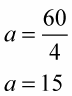##### Linear Algebra For Dummies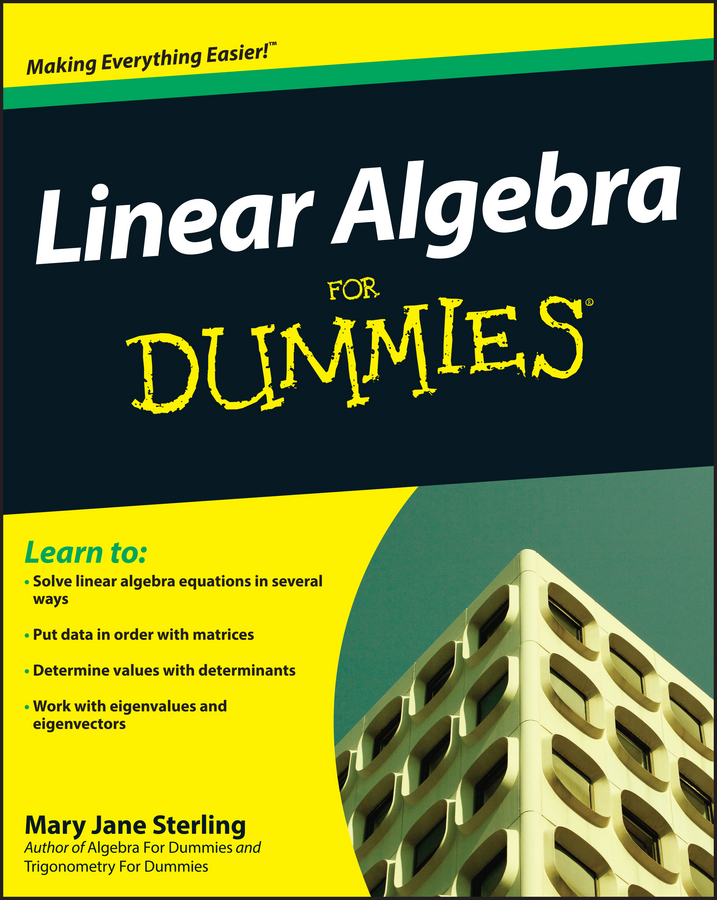Often, you need to use both multiplication and division to solve a linear equation. When a linear equation uses both multiplication and division, you solve by using the inverse operation of each. So, if a variable is both multiplied and divided by a number in an equation, you solve the equation for that variable by using a combination of multiplication and division.

Example: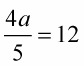Apply these steps to solve an equation with multiplication and division by trying to solve for a.

1. Determine the value that divides the variable.

In this case, the 5 is dividing into both the 4 and the variable.

2. Multiply the values on each side of the equal sign.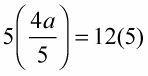3. Reduce and simplify.

The 5 cancels itself out on the left side of the equation, leaving you with

4a = 12(5)

4a = 60

4. Determine what is multiplying the variable.

The number 4 is the coefficient and multiplies the a.

5. Divide the values on each side of the equal sign.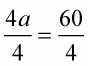6. Reduce and simplify.

The 4 cancels itself out on the left side of the equation, leaving you with